# Math test

Students wrote a math test. The average number of points obtained by them was 64. Another student wrote this test for 80 points. If the teacher added his result to the original ones, the total average of all students would be 65. How many students originally wrote the test?

n =  15

### Step-by-step explanation:

64n + 80 = 65•(n+1)

64•n + 80 = 65•(n+1)

n = 15

Our simple equation calculator calculates it.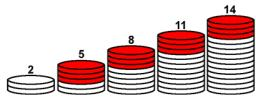Did you find an error or inaccuracy? Feel free to write us. Thank you!Tips to related online calculators
Looking for help with calculating arithmetic mean?
Looking for a statistical calculator?
Do you have a linear equation or system of equations and looking for its solution? Or do you have a quadratic equation?

#### You need to know the following knowledge to solve this word math problem:

We encourage you to watch this tutorial video on this math problem:

## Related math problems and questions:

• Average score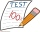In a math exam, two students scored 95, six students scored 90, 3 students scored 80, and one students scored 50. What was the average score for the class?
• Confidence Interval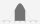The average grade of 50 students senior students in a statistics class is 85 with a standard deviation of 10.2 while a group of 60 senior students got an average of 80 with a standard deviation of 8.9, can the difference in the mean grade be attributed to
• Average height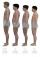In a class are 34 students. The average height of the students is 165 cm. What will be the average height of students in the classroom when two pupils, tall 176 cm and 170 cm, moved from this school/class? The average height in meters of all the students
• TestsTo obtain a grade A, Patrick must score an average of at least 70 in five tests. If his average mark for the four tests is 68, what is the lowest mark he can get in his fifth test and still obtain a grade A
• Average speed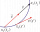The car drove on one section of the highway for half an hour at a speed of 80 km/h. Then he reduced his speed to 60km/h. It went three-quarters of an hour at a speed of 60 km/h. What was the average speed of the car?
• Contestants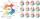In the competition it was possible to get 0-5 points. In fact, 5 contestants of the 15 best contestants has earned 5 points or 4 points (which have won 10 contestants). The number of contestants who earned 3 points was the same as the number of contestant
• Language testYour language test scores are 58, 68, 89 and 95. What is the lowest you can earn on the next test and still achieve an average of at least 79?
• TestingStudents of high school have 10 points for each good solved task. The wrong answer is deducted by 5 points. After solving 20 tasks, student Michael had 80 points. How many tasks did he solve correctly and how many wrong?
• Average heightThe average height of all pupils is 162 cm. The class teacher's height is 178 cm. The average height of all (teacher and all pupils) is 163 cm. Calculate the number of pupils in the class.
• 9.A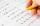9.A to attend more than 20 students but fewer than 40 students. A third of the pupils wrote a math test to mark 1, the sixth to mark 2, the ninth to mark 3. No one gets mark 4. How many students of class 9.A wrote a test to mark 5?
• Annual incomeThe annual income (in thousands of \$) of fifteen families is 60, 80, 90, 96, 120, 150, 200, 360, 480, 520, 1060, 1200, 1450, 2500, 7200. Calculate the harmonic and geometric mean.
• Petra's testThe answer will be given 8 marks, and the wrong answer will be deducted 3 marks. The total number of marks obtained by the school is 36. If Petra answered six questions correctly,   how many questions were answered incorrectly by the student from the scho
• A professorA professor in a typing class found out that the average performance of an expert typist is 85 words per minute. A random sample of 16 students took the typing test and an average speed of 62 words per minute was obtained with a standard deviation of 8. C
• Marks at schoolThere are 30 students in the class. Five students in the class had a mark three triple at the end of the math certificate, and the other students had a mark of one or two. The average mark in all students' mathematics in the class at the end of the year w
• FlyingThe airplane from Prague to Bratislava was flying at a speed of 60 km/h less and back by 70 km/h greater than the original speed. What was the original speed if the plane returned to Prague according to the timetable?
• Chess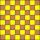How many ways can select 4 fields on a classic chessboard with 64 fields so that fields don't have the same color?
• Calf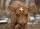The weight of the calf meat obtained is 65% of the total weight of live calves. What was the weight of a calf from which 285 kg of meat was obtained?